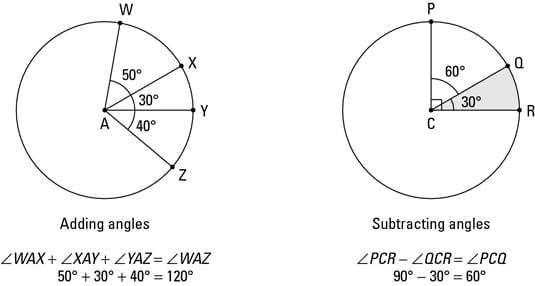##### Trigonometry For DummiesAdding and subtracting segments and angles isn’t exactly rocket science. But it is important because this geometric arithmetic comes up in proofs and other geometry problems. Here’s how it works:

• Adding and subtracting segments: To add or subtract segments, simply add or subtract their lengths. For example, if you put a 4-inch stick end-to-end with an 8-inch stick, you get a total length of 12 inches. That’s how segments add. Subtracting segments is like cutting off 3 inches from a 10-inch stick. You end up with a 7-inch stick. Brain surgery this is not.• Adding and subtracting angles: To add or subtract angles, you just add or subtract the angles’ degrees. You can think of adding angles as putting two or more pizza slices next to each other with their pointy ends together. Subtracting angles is like starting with some pizza and taking a piece away. The above figure shows how this works.